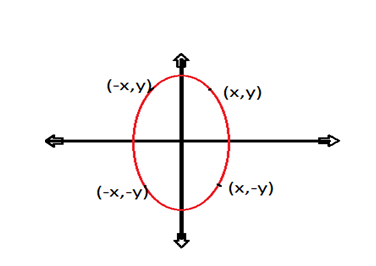# Ellipse Algorithm | Computer Graphics

In this article, we are going to learn about Ellipse generating algorithms in computer graphics i.e. Midpoint ellipse algorithm. Properties of ellipse are also prescribed in this article.
Submitted by Abhishek Kataria, on August 25, 2018

## Properties of ellipse

Ellipse is defined as the locus of a point in a plane which moves in a plane in such a manner that the ratio of its distance from a fixed point called focus in the same plane to its distance from a fixed straight line called directrix is always constant, which should always be less than unity.

If the distance to the two foci from any point P=(x,y) on the ellipse are labeled d1 and d2 then the general equation of the ellipse can be stated as- d1+d2=constant.

For expressing the distances d1 and d2 in terms of focal coordinates F1 and F2 we have:- Ax2+By2+Cxy+Dx+Ey+F=0 where A, B, C, D,E, and F are evaluated in terms of focal coordinates and dimensions of the major and minor axes of the ellipse.

## Midpoint ellipse algorithm

The midpoint ellipse method is applied throughout the first quadrant in two parts. Now let us take the start position at (0,ry) and step along the ellipse path in clockwise order throughout the first quadrant.

Ellipse function can be defined as:

fellipse(x,y)=ry2x2+rx2y2-rx2ry2

According to this there are some properties which have been generated that are:

1. fellipse(x,y)<0 which means (x,y) is inside the ellipse boundary.
2. fellipse(x,y)>0 which means (x,y) is outside the ellipse boundary.
3. fellipse(x,y)=0 which means (x,y) is on the ellipse boundary.### Initial decision parameter

In region 1 the initial value of a decision parameter is obtained by giving starting position = (0,ry).

i.e. p10=ry2+1/4rx2-rx2ry

When we enter into a region 2 the initial position is taken as the last position selected in region 1 and the initial decision parameter in region 2 is then:

p20=ry2(x0+1/2)2+rx2(y0-1)2-rx2ry2

### ALGORITHM

1. Take the input and ellipse centre and obtain the first point on an ellipse centered on the origin as a (x,y0)= (0,ry).
2. Now calculate the initial decision parameter in region 1 as:
p10=ry2+1/4rx2-rx2ry
3. At each xk position in region 1 perform the following task. If p1k<0 then the next point along the ellipse centered on (0,0) is (xk+1,yk).
i.e. p1k+1=p1k+2ry2xk+1+ry2
Otherwise the next point along the circle is (xk+1,yk -1)
i.e. p1k+1=p1k+2ry2xk+1 – 2rx2yk+1+ry2
4. Now, again calculate the initial value in region 2 using the last point (x0,y0) calculated in a region 1 as : p20=ry2(x0+1/2)2+rx2(y0-1)2-rx2ry2
5. At each yk position in region 2 starting at k =0 perform the following task. If p2k<0 the next point along the ellipse centered on (0,0) is (xk , yk-1)
i.e. p2k+1=p2k-2rx2yk+1+rx2
Otherwise the next point along the circle will be (xk+1,yk -1)
i.e. p2k+1 =p2k+2ry2xk+1 -2rx2yk+1+rx2
6. Now determine the symmetric points in another three quadrants.
7. Plot the coordinate value as: x=x+xc , y=y+yc
8. Repeat the steps for region 1 until 2ry2x>=2rx2y.